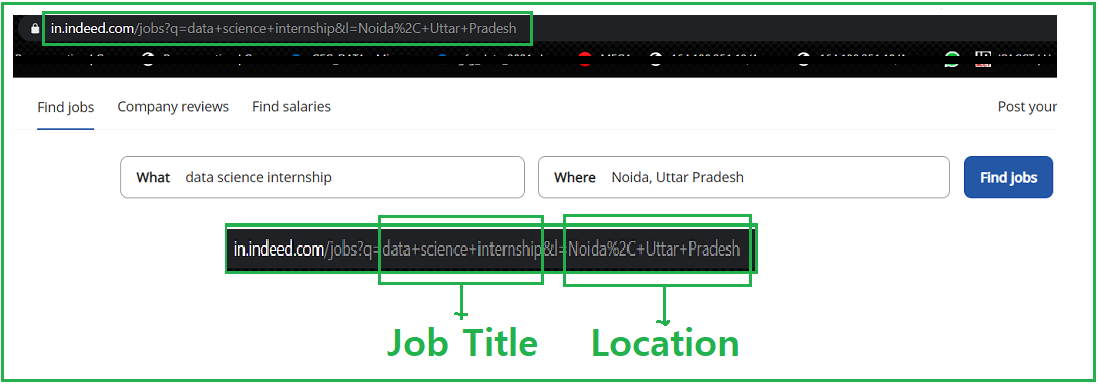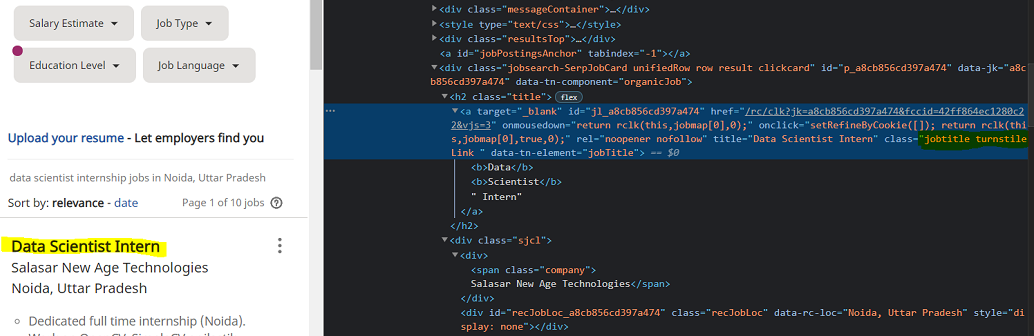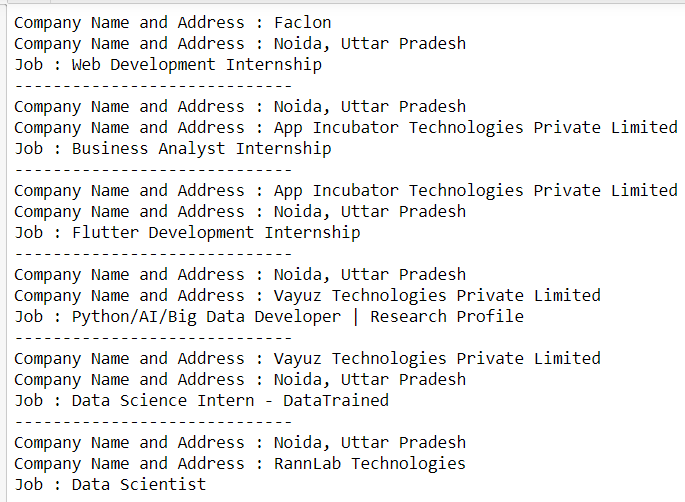# Scraping Indeed Job Data Using Python

In this article, we are going to see how to scrape Indeed job data using python. Here we will use Beautiful Soup and the request module to scrape the data.

### Module needed

• bs4: Beautiful Soup(bs4) is a Python library for pulling data out of HTML and XML files. This module does not come built-in with Python. To install this type the below command in the terminal.

pip install bs4

• requests: Request allows you to send HTTP/1.1 requests extremely easily. This module also does not come built-in with Python. To install this type the below command in the terminal.

pip install requests

### Approach:

• Import all the required modules.
• Pass the URL in the getdata() function(User Defined Function) to that will request to a URL, it returns a response. We are using get method to retrieve information from the given server using a given URL.

Syntax:

requests.get(url, args)

• Convert that data into HTML code.

In the given image we see the link, where we search the job and its location then the URL becomes something like this https://in.indeed.com/jobs?q=”+job+”&l=”+Location, Hence we will format our string into this format.• Now Parse the HTML content using bs4.

Syntax: soup = BeautifulSoup(r.content, ‘html5lib’)

Parameters:

• r.content : It is the raw HTML content.
• html.parser : Specifying the HTML parser we want to use.
• Now filter the required data using soup.Find_all function.
• Now find the list with a tag where class_ = jobtitle turnstileLink. You can open the webpage in the browser and inspect the relevant element by pressing right-click as shown in the figure.• Find the Company name and address with the same as the above methods.

### Functions used:

The code for this implementation is divided into user defined functions to increase the readability of the code and add ease of use.

• geturl(): gets the URL from which data is to be scraped
• html_code(): get HTML code of the URL provided
• job_data(): filter out job data
• Company_data(): filter company data

Program:

## Python3

 `# import module ` `import` `requests ` `from` `bs4 ``import` `BeautifulSoup ` ` `  ` `  `# user define function ` `# Scrape the data ` `# and get in string ` `def` `getdata(url): ` `    ``r ``=` `requests.get(url) ` `    ``return` `r.text ` ` `  `# Get Html code using parse ` `def` `html_code(url): ` ` `  `    ``# pass the url ` `    ``# into getdata function ` `    ``htmldata ``=` `getdata(url) ` `    ``soup ``=` `BeautifulSoup(htmldata, ``'html.parser'``) ` ` `  `    ``# return html code ` `    ``return``(soup) ` ` `  `# filter job data using ` `# find_all function ` `def` `job_data(soup): ` `   `  `    ``# find the Html tag ` `    ``# with find() ` `    ``# and convert into string ` `    ``data_str ``=` `"" ` `    ``for` `item ``in` `soup.find_all(``"a"``, ``class_``=``"jobtitle turnstileLink"``): ` `        ``data_str ``=` `data_str ``+` `item.get_text() ` `    ``result_1 ``=` `data_str.split(``"\n"``) ` `    ``return``(result_1) ` ` `  `# filter company_data using ` `# find_all function ` ` `  ` `  `def` `company_data(soup): ` ` `  `    ``# find the Html tag ` `    ``# with find() ` `    ``# and convert into string ` `    ``data_str ``=` `"" ` `    ``result ``=` `"" ` `    ``for` `item ``in` `soup.find_all(``"div"``, ``class_``=``"sjcl"``): ` `        ``data_str ``=` `data_str ``+` `item.get_text() ` `    ``result_1 ``=` `data_str.split(``"\n"``) ` ` `  `    ``res ``=` `[] ` `    ``for` `i ``in` `range``(``1``, ``len``(result_1)): ` `        ``if` `len``(result_1[i]) > ``1``: ` `            ``res.append(result_1[i]) ` `    ``return``(res) ` ` `  ` `  `# driver nodes/main function ` `if` `__name__ ``=``=` `"__main__"``: ` ` `  `    ``# Data for URL ` `    ``job ``=` `"data+science+internship"` `    ``Location ``=` `"Noida%2C+Uttar+Pradesh"` `    ``url ``=` `"https://in.indeed.com/jobs?q="``+``job``+``"&l="``+``Location ` ` `  `    ``# Pass this URL into the soup ` `    ``# which will return ` `    ``# html string ` `    ``soup ``=` `html_code(url) ` ` `  `    ``# call job and company data ` `    ``# and store into it var ` `    ``job_res ``=` `job_data(soup) ` `    ``com_res ``=` `company_data(soup) ` ` `  `    ``# Traverse the both data ` `    ``temp ``=` `0` `    ``for` `i ``in` `range``(``1``, ``len``(job_res)): ` `        ``j ``=` `temp ` `        ``for` `j ``in` `range``(temp, ``2``+``temp): ` `            ``print``(``"Company Name and Address : "` `+` `com_res[j]) ` ` `  `        ``temp ``=` `j ` `        ``print``(``"Job : "` `+` `job_res[i]) ` `        ``print``(``"-----------------------------"``) `

Output:Whether you're preparing for your first job interview or aiming to upskill in this ever-evolving tech landscape, GeeksforGeeks Courses are your key to success. We provide top-quality content at affordable prices, all geared towards accelerating your growth in a time-bound manner. Join the millions we've already empowered, and we're here to do the same for you. Don't miss out - check it out now!